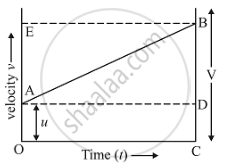# Derive the Following Equation of Motion by the Graphical Method : V2 = U2 + 2as, Where the Symbols Have Their Usual Meanings. - Science

Derive the following equation of motion by the graphical method : v2 = u2 + 2as, where the symbols have their usual meanings.

#### SolutionIn the given figure, the distance travelled (s)  by a body in time  (t) is given by the area of the figure OABC which is a trapezium.
Distance travelld = Area of the trapezium OABC
So, Area of trapezium OABC,
= "(Sum of parallel sides)(Height)"/2
="(OA+CB)(OC)"/2
Now, (OA + CB) = u + v and (OC) = t.
Putting these values in the above relation, we get:
s = ((u+v)/2)t ....(1)
Eliminate t from the above equation. This can be done by obtaining the value of t from the first equation of motion.
v = u + at
So,
t = "v-u"/a
Now, put this value of t in equation (1), we get:
s = (((u+v)(v-u))/(2a))
On further simplification,
2as = v2 – u2
Finally the third equation of motion.
v^2 = u^2 + 2as
where
(s) - Displacement
(u) - Initial velocity
(a) - Acceleration
(v) - Final velocity
(t) - Time taken

Concept: Velocity - Time Graphs
Is there an error in this question or solution?

#### APPEARS IN

Lakhmir Singh Science Class 9 Physics
Chapter 1 Motion
Long Answers | Q 40 | Page 42